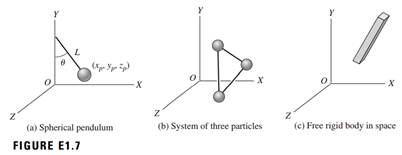### Create an Account

Already have account?

### Forgot Your Password ?

Home / Questions / Determine the number of degrees of freedom for the systems shown in Figure E17 Assume that...

# Determine the number of degrees of freedom for the systems shown in Figure E17 Assume that the length L of the pendulum shown in Figure E1 7a is constant and that the length between each pair of

Determine the number of degrees of freedom for the systems shown in Figure E1.7. Assume that the length L of the pendulum shown in Figure E1.7a is constant and that the length between each pair of particles in Figure E1.7b is constant.May 16 2020 View more View Less

#### Answer (Solved)Subscribe To Get Solution